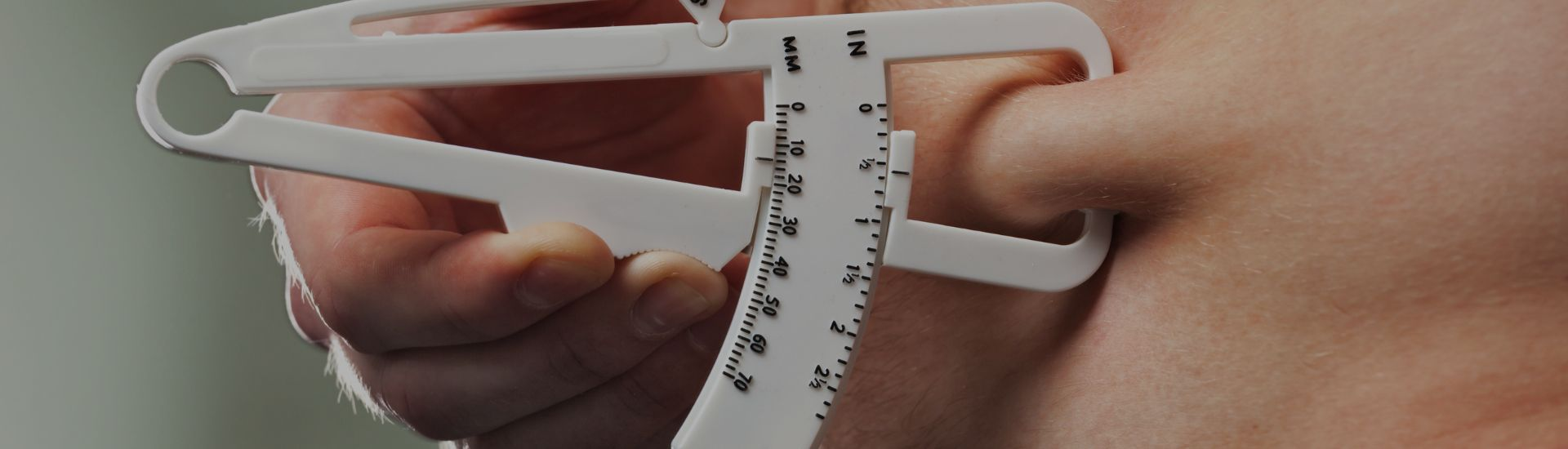TOP# Katch McArdle Equation for nutrition professionals

The body constantly uses energy to be able to breathe, circulate blood, and even to ensure the proper functioning of the brain and its adjacent functions. These energy uses are considered the "resting metabolism", the basal metabolic rate (BMR). Knowing an individual's BMR is essential to know how many calories should be ingested daily, so in this article we will address the Katch McArdle equation.

The Katch McArdle equation is a predictive equation for resting/basal energy expenditure in healthy individuals. Learn all about it in our article.

## The origin of the Katch MacArdle equation

Frank I. Katch and William D. McArdle conducted a study to evaluate the validity of body composition prediction equations for college men and women. They measured body density, using the water submersion technique, and body composition, using hydrostatic weighing. Fat is lighter than bone and muscle, so its density can be used to calculate the percentage of body fat. And in this way, reach the predictive equation.

The study was done on 136 college men and women at Queens College, New York, and was published in 1975.

The authors compared the measured values with the values predicted by the prediction equations. They found that the prediction equations were more accurate for men than for women. The study concluded that body composition prediction equations can be useful for college men and women, but it is important to validate the equations in different samples before using them in other studies.

### Did you know that…

… when conducting the study, the researchers also learned that the prediction equation based on waist circumference was more accurate for men and the prediction equation based on hip circumference was more accurate for women.

## When should you use the Katch McArdle equation?

This equation is useful when it is necessary to calculate BMR in healthy young adult individuals and when you have access to lean body mass values. It is not necessary to know age, height, or gender.

This is also a validated equation for athletes, especially those with high lean body mass.

## What sets it apart from other predictive equations for basal energy expenditure?

This equation takes into account an individual's lean body mass (fat-free mass), whereas most other equations consider only body weight, height, age, and gender

This is important because lean body mass is a better indicator of an individual's metabolic rate than total body weight, since muscle tissue is metabolically more active than fat tissue. Therefore, the Katch-McArdle equation is considered more accurate for individuals with low percentages of body fat or high amounts of muscle mass, such as athletes.

## When to use this equation?

It is indicated for clients who exercise, in the age range of 17 to 24. It does not differentiate between gender, ethnicity, or age.

## How to calculate the BMR?

### - Calculate lean body mass

Subtract body fat from body weight. It is important to know the amount of fat, since muscle requires more energy than body fat to function properly.

(1 - 0.% body fat) x Kg weight = lean body mass

Eg..: 75 kg and 28% body fat

(1-0,28) x 75 = 54 kg of lean body mass

### - Katch Mcardle Equation

370 + (9,82 x lean body mass)

Eg.: 370 + (9,82 x 54) = 900,28 BMR

### - Multiply by the level of activity

Sedentary - BMR X 1,2 (little to no exercise, desk job)

Light Activity - BMR X 1.375 (exercise 1 to 3 days per week)

Moderate Activity - BMR X 1,55 (exercise 3 to 5 days per week)

Very Active - BMR X 1.725 (exercise 6 to 7 dias per week)

Extremely Active - BMR X 1,9 (exercise 2x a day)

Eg.: Light Activity (1,375)

900,28 x 1,375 = 1237,885 BMR

## References

Validity of body composition prediction equations for college men and women